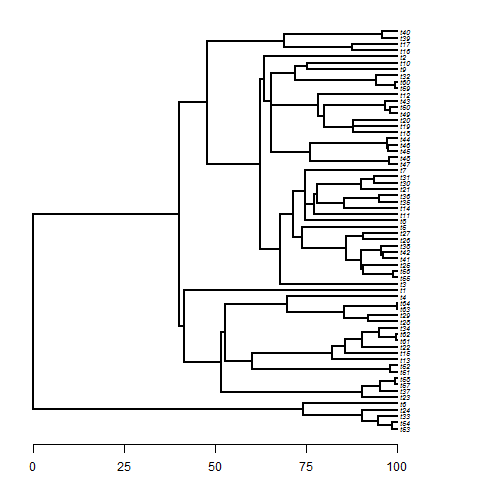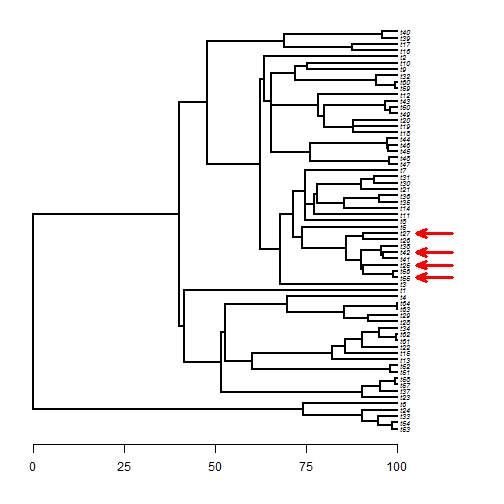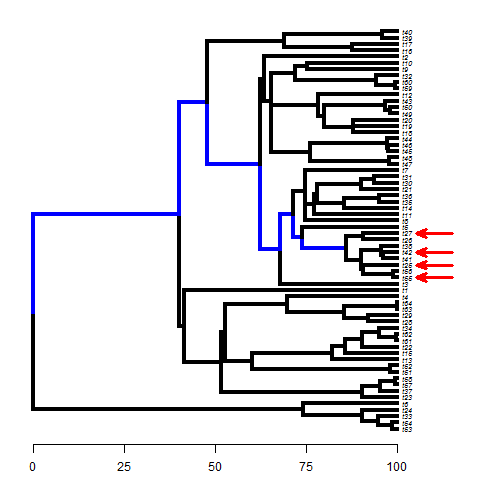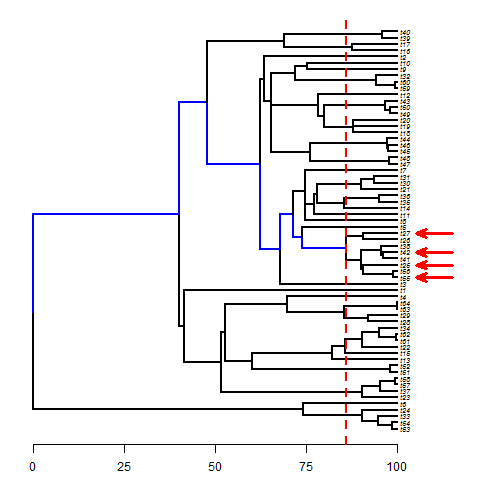## Wednesday, October 11, 2017

### Finding the amount of history (branch length) shared among the members of a set of taxa

Today I received the following inquiry from a friend in Chile:

“I want to get the shared branch lengths for more than two species. I can get this information but just for two species using the `vcv` function a other like it. Can you help me with this?”

This would not be too difficult to do using `getMRCA` and either the phytools function `vcvPhylo` or `nodeheight`.

So, if we imagine the following tree:

``````library(phytools)
library(phangorn)
plotTree(tree,fsize=0.6,ftype="i",
mar=c(4.1,1.1,1.1,1.1),xlim=c(0,120))
axis(1,at=seq(0,100,by=25))
``````and what we want is the shared history from the root to the MRCA of (say) the four taxa t55, t25, t42, and t27 (though the following code works for an arbitrary number of taxa):

``````plotTree(tree,fsize=0.6,ftype="i",
mar=c(4.1,1.1,1.1,1.1),xlim=c(0,120))
axis(1,at=seq(0,100,by=25))
tips<-c("t55","t25","t42","t27")
arrl=10,lwd=3,lend=0,offset=2)
``````what we are after is the sum of the edges shown in blue below:

``````ANCESTORS<-lapply(sapply(tips,function(x,y) which(y==x),y=tree\$tip.label),
Ancestors,x=tree,type="all")
edges<-setdiff(Reduce(intersect,ANCESTORS),Ntip(tree)+1)
plot(paintBranches(tree,edges,"2"),fsize=0.6,ftype="i",
mar=c(4.1,1.1,1.1,1.1),xlim=c(0,120),
colors=setNames(c("black","blue"),1:2),
lwd=4,split.vertical=TRUE)
axis(1,at=seq(0,100,by=25))
arrl=10,lwd=3,lend=0,offset=2)
``````We can get this using `vcvPhylo` as follows:

``````h<-vcvPhylo(tree)[as.character(getMRCA(tree,tips)),
as.character(getMRCA(tree,tips))]
h
``````
``````##  85.96251
``````
``````plot(paintBranches(tree,edges,"2"),fsize=0.6,ftype="i",
mar=c(4.1,1.1,1.1,1.1),xlim=c(0,120),
colors=setNames(c("black","blue"),1:2),
lwd=2,split.vertical=TRUE)
axis(1,at=seq(0,100,by=25))
arrl=10,lwd=3,lend=0,offset=2)
abline(v=h,col="red",lwd=2,lty="dashed")
``````Or we could use `nodeheight` in a similar way:

``````h<-nodeheight(tree,getMRCA(tree,tips))
h
``````
``````##  85.96251
``````
``````plot(paintBranches(tree,edges,"2"),fsize=0.6,ftype="i",
mar=c(4.1,1.1,1.1,1.1),xlim=c(0,120),
colors=setNames(c("black","blue"),1:2),
lwd=2,split.vertical=TRUE)
axis(1,at=seq(0,100,by=25))
arrl=10,lwd=3,lend=0,offset=2)
abline(v=h,col="red",lwd=2,lty="dashed")
``````However, though this is easy enough, the function `findMRCA` also has an option `type="height"` which returns exactly the same quantity! It works as follows:

``````h<-findMRCA(tree,tips,type="height")
h
``````
``````##  85.96251
``````

and that's all there is to it!

Note that there is no requirement that our taxa form a clade - though of course they can.